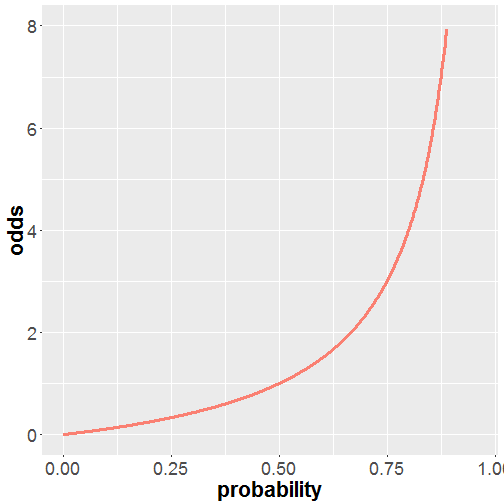# Introduction to Open Data Science

University of Helsinki, spring 2017

• Tuomo Nieminen and Emma Kämäräinen with

From data wrangling to exploration and modelling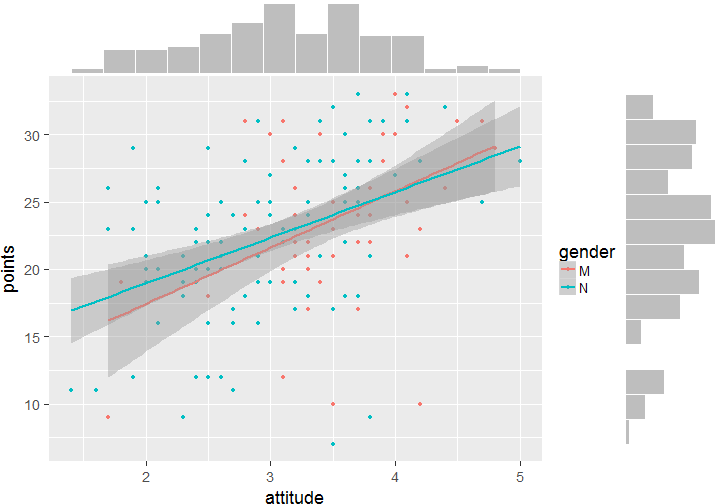### Contents

1. Regression and model validation
2. Logistic regression
3. Clustering and classification
4. Dimensionality reduction techniques

## Linear regression and model validation

For IODS by Tuomo Nieminen

### Linear regression models

Simple regression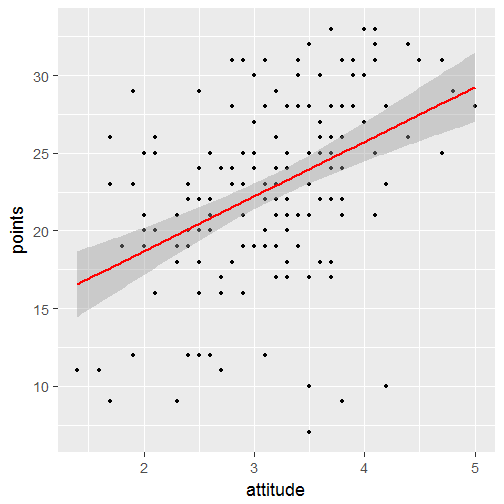Multiple regression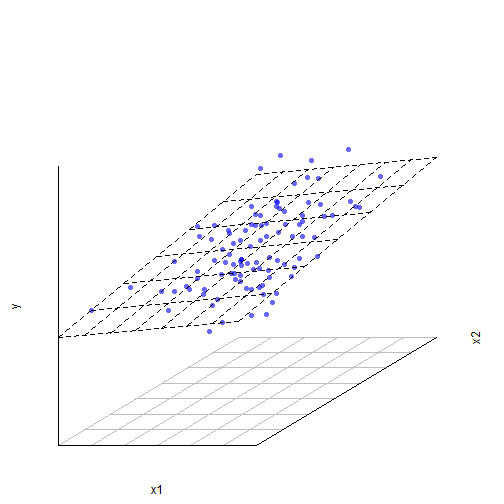### What is a statistical model?

A statistical model:

• Embodies a set of assumptions and describes the generation of a sample from a population
• Represents the data generating process
• The uncertainty related to a sample of data is described using probability distributions

### Linear regression models

Linear regression is an approach for modeling the relationship between a dependent variable $$\boldsymbol{y}$$ and one or more explanatory variables $$\boldsymbol{X}$$.

There are many applications for linear models such as

• Prediction or forecasting
• Quantifying the strength of the relationship between $$\boldsymbol{y}$$ and $$\boldsymbol{x}$$

### Simple regression

In a simple case, the model includes one explanatory variable $$\boldsymbol{x}$$

$$\boldsymbol{y} = \alpha + \beta \boldsymbol{x} + \boldsymbol{\epsilon}$$

R:
lm(y ~ x)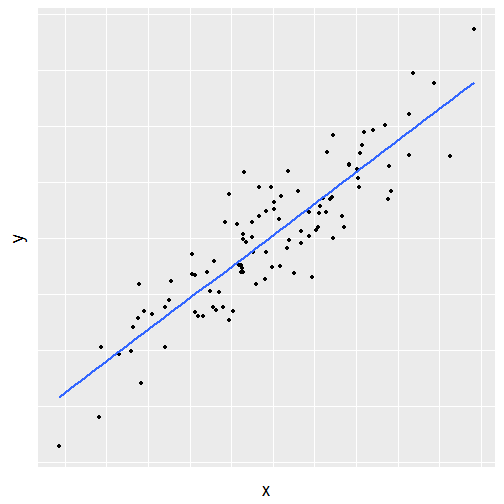### Multiple regression

The model can also include more than one explanatory variable

$\boldsymbol{y} = \alpha + \beta_1 \boldsymbol{x}_1 + \beta_2 \boldsymbol{x}_2 + \boldsymbol{\epsilon}$

R:
lm(y ~ x1 + x2)### Assumptions of linear regression models

In linear regression, it is assumed that the relationship between the target variable $$\boldsymbol{y}$$ and the parameters ($$\alpha$$, $$\boldsymbol{\beta}$$) is linear:

$\boldsymbol{y} = \boldsymbol{\alpha} + \boldsymbol{X} \boldsymbol{\beta} + \boldsymbol{\epsilon}$

• The goal is to estimate the parameters $$\alpha$$ and $$\boldsymbol{\beta}$$, which describe the relationship with the explanatory variables $$\boldsymbol{X}$$
• An unobservable random variable ($$\boldsymbol{\epsilon}$$) is assumed to add noise to the observations
• Often it is reasonable to assume $$\boldsymbol{\epsilon} \sim N(0, \sigma^2)$$

### Structure of a linear model

In the simple linear equation $$\boldsymbol{y} = \alpha + \beta \boldsymbol{x} + \boldsymbol{\epsilon}$$

• $$\boldsymbol{y}$$ is the target variable: we wish to predict the values of $$\boldsymbol{y}$$ using the values of $$\boldsymbol{x}$$.
• $$\alpha + \beta \boldsymbol{x}$$ is the systematic part of the model.
• $$\beta$$ quantifies the relationship between $$\boldsymbol{y}$$ and $$\boldsymbol{x}$$.
• $$\boldsymbol{\epsilon}$$ describes the errors (or the uncertainty) of the model

### Finding the model

The best model is found by minimizing the prediction errors that the model would make

• $$\hat{\boldsymbol{y}} = \hat{\alpha} + \hat{\beta} \boldsymbol{x}$$ are the predictions
• $$\boldsymbol{\hat{\epsilon}} = \hat{\boldsymbol{y}} - \boldsymbol{y}$$ are the prediction errors, called residuals
• The model is found by minimizing the sum of squared residuals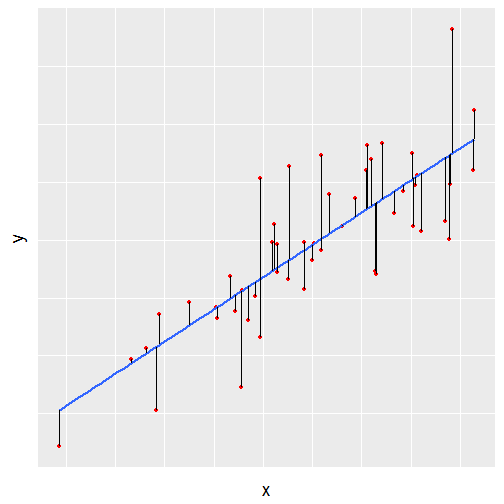### Interpreting the parameters

When the model is $\boldsymbol{y} = \alpha + \beta_1 \boldsymbol{x}_1 + \beta_2 \boldsymbol{x}_2 + \boldsymbol{\epsilon}$

• The main interest is to estimate the $$\boldsymbol{\beta}$$ parameters
• Interpretation of an estimate $$\hat{\beta_1} = 2$$:
• When $$x_1$$ increases by one unit, the average change in $$y$$ is 2 units, given that the other variables (here $$x_2$$) do not change.

### R linear model summary()

For a quick rundown of interpreting R's regression summary, see the 'Calling summary' section of this blog post or read about coefficients and p-values here


Call:
lm(formula = Y ~ some_variable)

Residuals:
Min      1Q  Median      3Q     Max
-5.2528 -1.8261 -0.1636  1.5288  5.8723

Coefficients:
Estimate Std. Error t value Pr(>|t|)
(Intercept)   -0.04364    0.49417  -0.088  0.93026
some_variable  1.81379    0.58925   3.078  0.00463 **
---
Signif. codes:  0 '***' 0.001 '**' 0.01 '*' 0.05 '.' 0.1 ' ' 1

Residual standard error: 2.643 on 28 degrees of freedom
Multiple R-squared:  0.2528,    Adjusted R-squared:  0.2262
F-statistic: 9.475 on 1 and 28 DF,  p-value: 0.004626


The linearity assumption isn't as restrictive as one could imagine.

It is possible to add polynomial terms to the model if the effect of a variable is non-linear

$\boldsymbol{y} = \alpha + \beta_1 \cdot \boldsymbol{x} + \beta_2 \cdot \boldsymbol{x}^2 + \boldsymbol{\epsilon}$

R:
lm(y ~ x + I(x^2))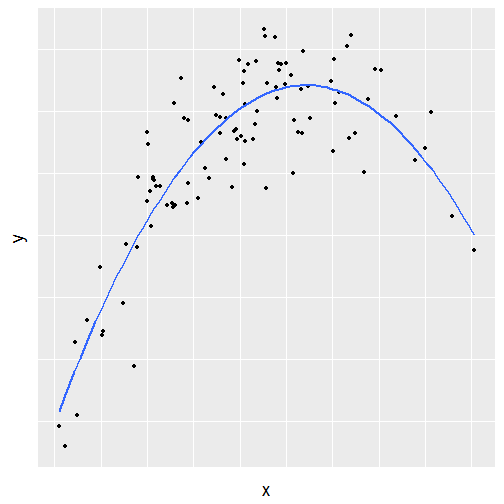### Model validation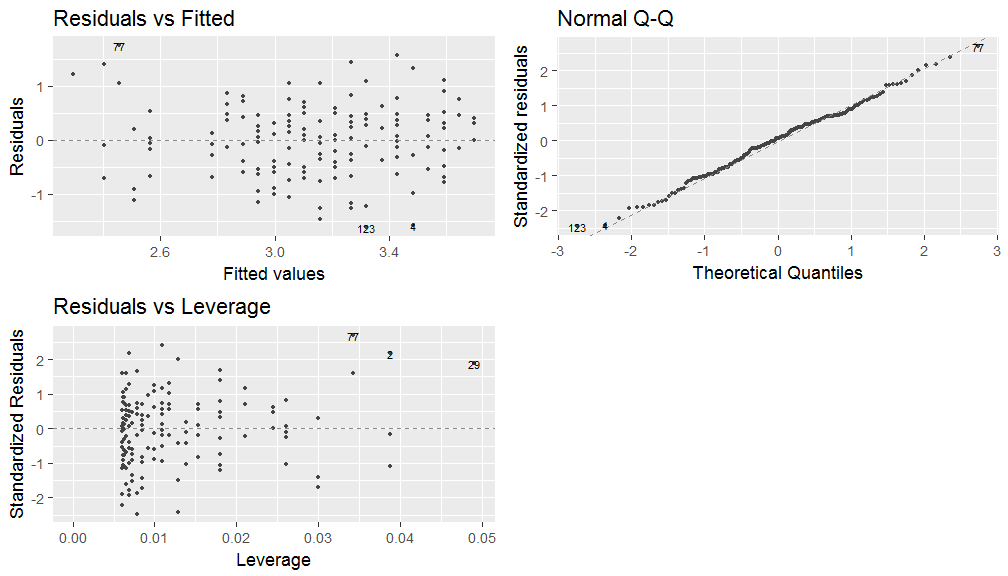### Model assumptions

A statistical model always includes several assumptions which describe the data generating process.

• How well the model describes the phenomenom of interest, depends on how well the assumptions fit reality.
• In a linear regression model an obvious assumption is linearity: The target variable is modelled as a linear combination of the model parameters.
• Usually it is assumed that the errors are normally distributed.

### Assumptions of linear regression models

Analyzing the residuals of the model provides a method to explore the validity of the model assumptions. A lot of interesting assumptions are included in the expression

$\boldsymbol{\epsilon} \sim N(0, \sigma^2)$

• The errors are normally distributed
• The errors are not correlated
• The errors have constant variance, $$\sigma^2$$
• The size of a given error does not depend on the explanatory variables

### Normality of the errors (QQ-plot)

QQ-plot of the residuals provides a method to explore the assumption that the errors of the model are normally distributed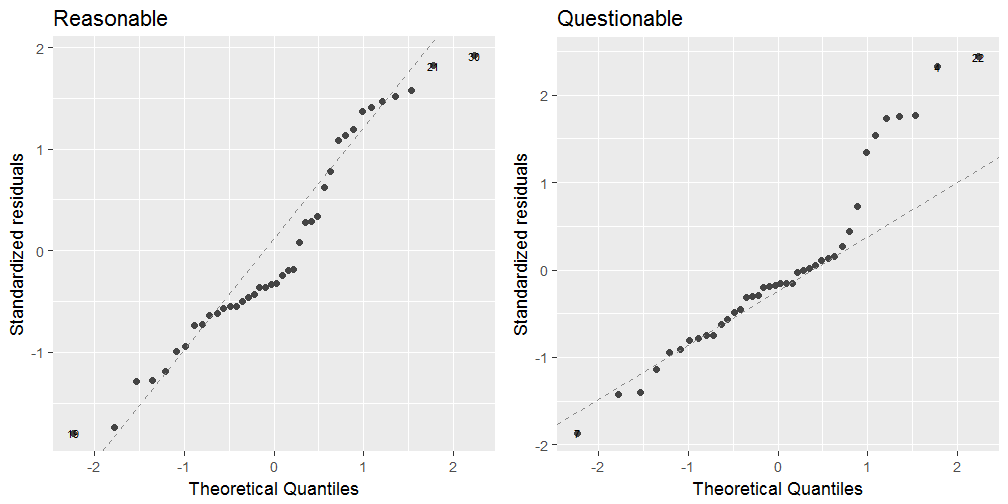### Constant variance of errors

The constant variance assumption implies that the size of the errors should not depend on the explanatory variables.

This can be explored with a simple scatter plot of residuals versus model predictions.

Any patter in the scatter plot implies a problem with the assumptions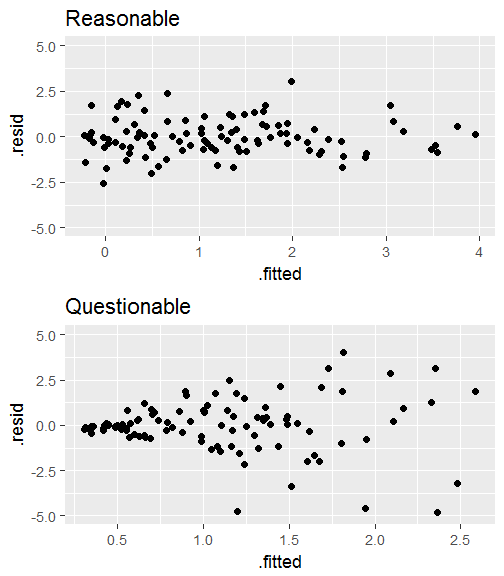### Leverage of observations (1)

Leverage measures how much impact a single observation has on the model.

• Residuals vs leverage plot can help identify which observations have an unusually high impact.
• The next two slides show four examples.
• Each row of two plots defines a data - model validation pair.

### Leverage of observations (2)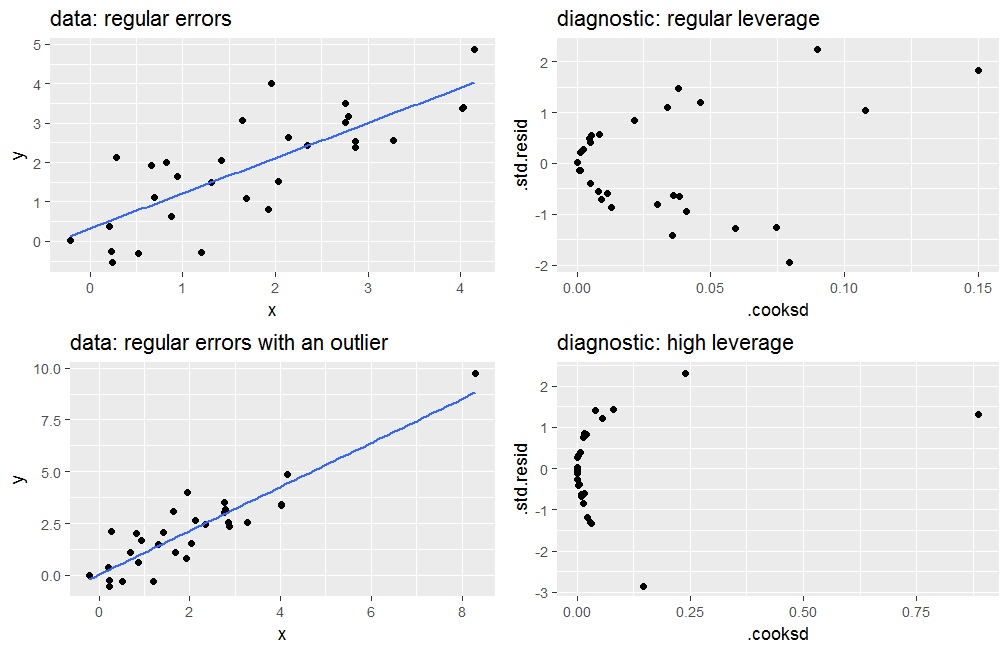### Leverage of observations (3)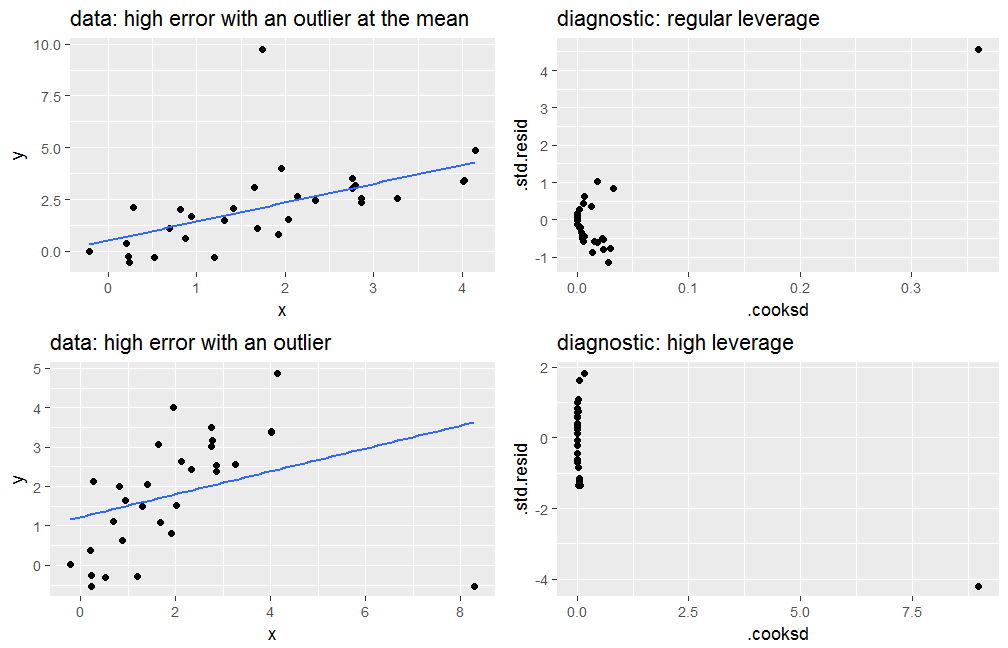## Logistic regression and cross-validation

For IODS by Tuomo Nieminen

Odds and probability